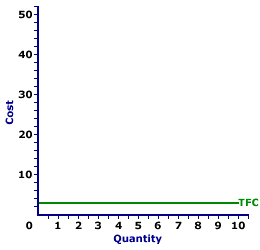Friday  December 8, 2023
 AmosWEB means Economics with a Touch of Whimsy!ENERGY PRICES, AGGREGATE SUPPLY DETERMINANT: One of several specific aggregate supply determinants assumed constant when the aggregate supply curve is constructed, and that shifts the aggregate supply curve when it changes. An increase in the energy prices causes a decrease (leftward shift) of the aggregate supply curve. A decrease in the energy prices causes an increase (rightward shift) of the aggregate supply curve. Other notable aggregate supply determinants include technology, wages, and the capital stock. Energy prices fall under the resource price aggregate supply determinant.TOTAL FIXED COST CURVE:

A curve that graphically represents the relation between total fixed cost incurred by a firm in the short-run product of a good or service and the quantity produced. This curve is constructed to capture the relation between total fixed cost and the level of output, holding other variables, like technology and resource prices, constant. Because total fixed cost are, in fact, fixed, the total fixed cost curve is, in fact, a horizontal line. The total fixed cost curve is one of three total cost curves, the other two are total cost curve and total variable cost curve.
Total Fixed Cost CurveThe total fixed cost curve is the most straightforward of the three total curves (the other two being total cost curve and total variable cost curve). The reason for such straightforwardness is that total fixed cost is fixed. It is the same at all output levels.

The total fixed cost curve can be derived in two ways. One is to plot a schedule of numbers relating output quantity and total fixed cost. The other is to vertically subtract the total variable cost curve from the total cost curve. The total fixed cost curve can be used to derive average fixed cost.

Total fixed cost is visually illustrated by the graph to the right, which is the total fixed cost curve for the short-run production of Wacky Willy Stuffed Amigos (those cute and cuddly armadillos and tarantulas). The quantity of Stuffed Amigos production, measured on the horizontal axis, ranges from 0 to 10 and the total fixed cost incurred in the production of Stuffed Amigos, measured on the vertical axis, is \$3 at each quantity of production.

Because total fixed cost is fixed, the total fixed cost curve is a horizontal line that intersects the vertical axis at \$3. If production is 0 Stuffed Amigos, total fixed cost is \$3. If Stuffed Amigo production is 10, total fixed cost is \$3. If production of Stuffed Amigos is boosted to a billion, then total fixed cost is \$3.

 <= TOTAL FIXED COST TOTAL-MARGINAL RELATION =>Recommended Citation:

TOTAL FIXED COST CURVE, AmosWEB Encyclonomic WEB*pedia, http://www.AmosWEB.com, AmosWEB LLC, 2000-2023. [Accessed: December 8, 2023].

Check Out These Related Terms...

Or For A Little Background...

And For Further Study...
Search Again?YELLOW CHIPPEROON[What's This?] Today, you are likely to spend a great deal of time strolling around a discount warehouse buying club trying to buy either decorative celebrity figurines or a flower arrangement with anything but tulips for your grandfather. Be on the lookout for defective microphones.Your Complete ScopeA communal society, a prime component of Karl Marx's communist philosophy, was advocated by the Greek philosophy Plato."If you don't know where you are going, any road will get you there."-- Lewis Carroll, writerFDICFederal Deposit Insurance CorporationA PEDestrian's Guide Xtra CreditTell us what you think about AmosWEB. Like what you see? Have suggestions for improvements? Let us know. Click the User Feedback link.| | | | | | | | | | |
| | | |

Thanks for visiting AmosWEB